Navigation: Software User Interface > Graphical Windows > Trade Plots and Analysis

In addition to individual trade review, much can be learned by analyzing all the trades of a test (or even a huge abstract sample of artificial trades) in the aggregate.

Aggregate trade level analysis works best in systems with 1000 or more trades.

To view a trade plot and access all the trade analysis features, right-click on the test and select "Show Trade Plots".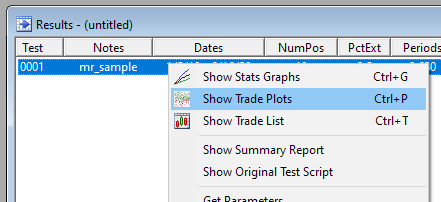The simplest kind of trade plot is the scatter plot: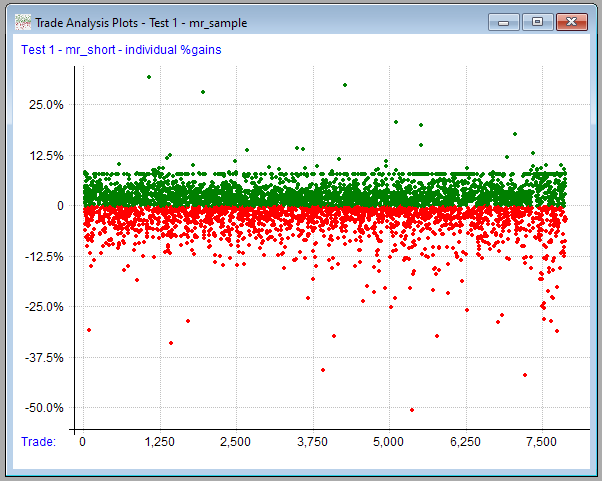This gives a feel for distribution of trade results.

To quantify the trade distribution a bit more, we can also view it this way: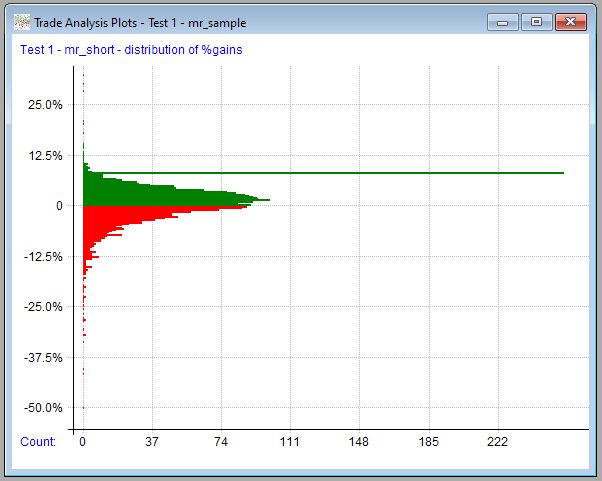(I know, histograms are usually displayed vertically ... I wanted to use the same axes as the scatter plot.)

Similar to the gain distribution plot is the MAE / MFE distribution: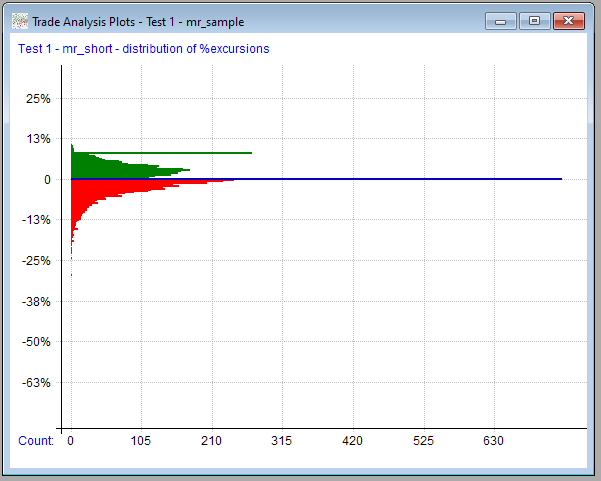Values of 0 (the most of any specific value in this example) are shown in blue. (Out of about 7000 trades, approximately 10% had no favorable excursion.)

The number of distribution bins to use in the above two plot types can be specified in the plot options dialog.

The same set of trades can also be plotted in a cumulative line with new highs marked and an optional linear regression line added: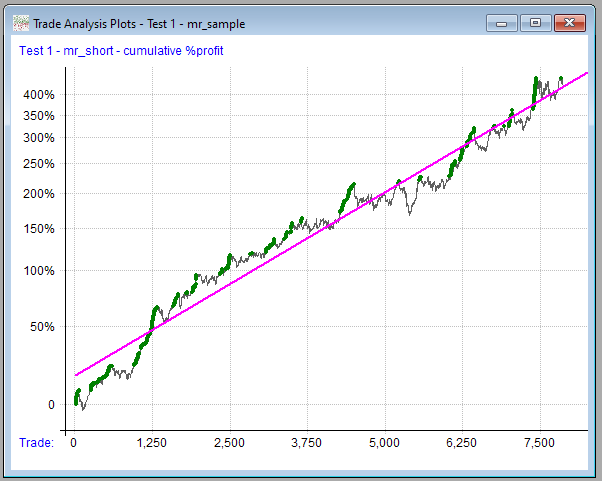This is the same set of dots as the first plot, with each one added to the prior one.

It will usually look something like the equity curve stats graph.

Next we have the Monte Carlo profit curves plot: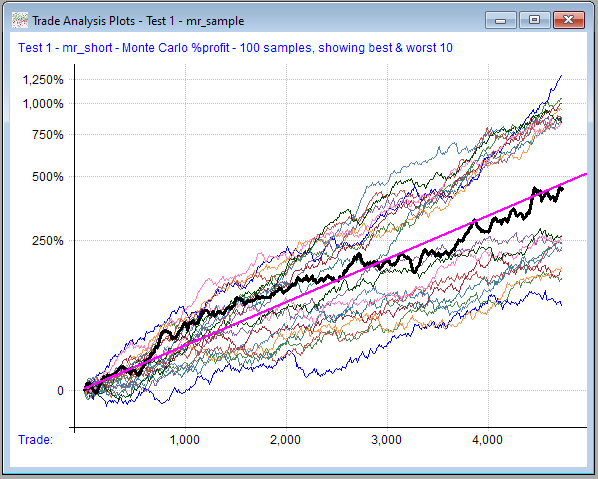In this case, 100 random cumulative profit curves were generated by taking random samples (with replacement) from the trade list.

The original backtest profit curve is plotted in bold black over them for comparison. A linear regression line is also plotted (magenta) after being calculated from all the combined random curves.

Next we have the Monte Carlo drawdown plot: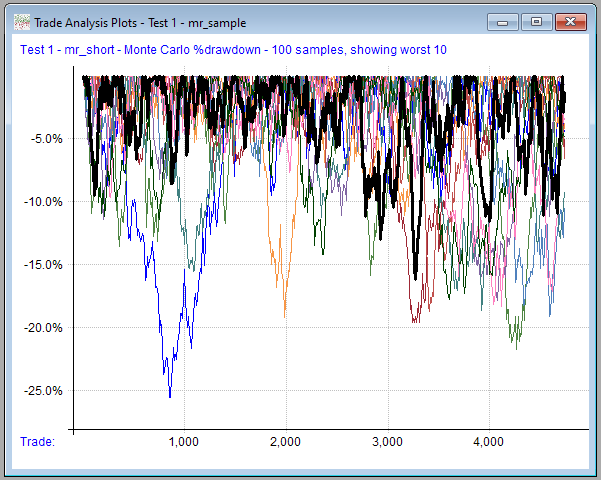RealTest can optionally create a table of Monte Carlo percentile stats and write it to the log window: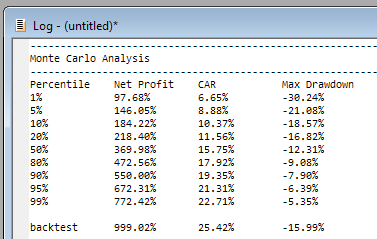To understand the other two plot types, we need to first visit the plot options dialog: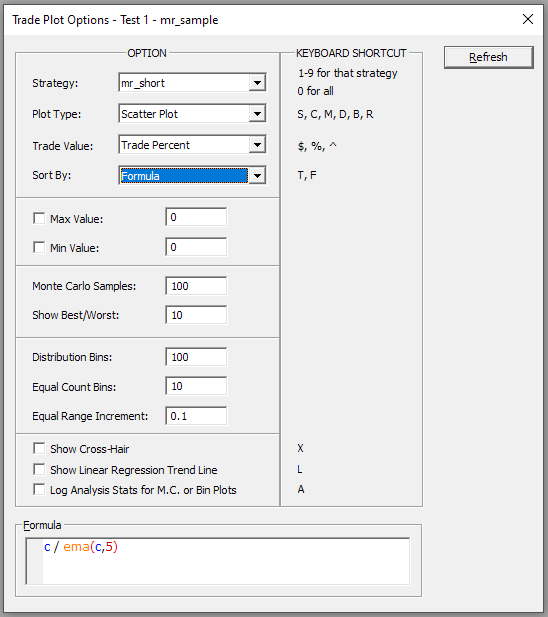Since this dialog also serves as a research tool, it has been made "modeless", meaning you can leave it open while using other windows. (Note that if plots are opened for more than one test at once, each plot will have its own options dialog window, with the test number and name shown in the caption.)

In any of the non-cumulative plot types, the trades can optionally be sorted using any criteria you want.

The range of values from the sort formula becomes the X Axis, while the Y Axis continues to show the range of trade profits.

Below, for example, we see the scatter plot of trades sorted by distance from the 5-period exponential moving average as of the day prior to entry (in this case, the "setup" day, since entry was the next day via limit order - the formula in this dialog is evaluated using the same "current bar" as the EntrySetup formula for each trade.)

Clicking "Refresh" produces the following plot: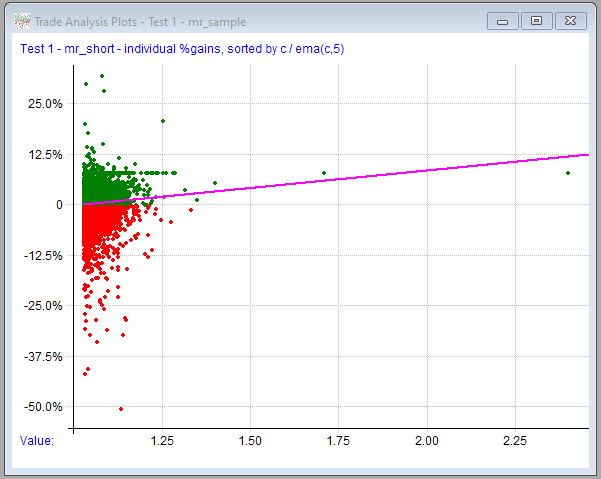This appears to show that there's some correlation between distance above a 5-day EMA on setup day and positive trade outcome.

Going a level deeper, let’s group the trades sorted by this formula into equal sized bins and calculate the expectancy of each bin.

Option settings: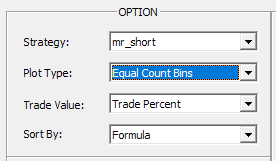...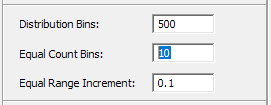Plot output: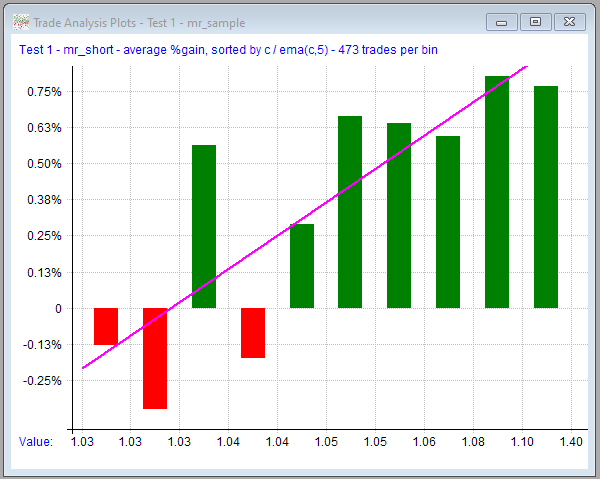Log output: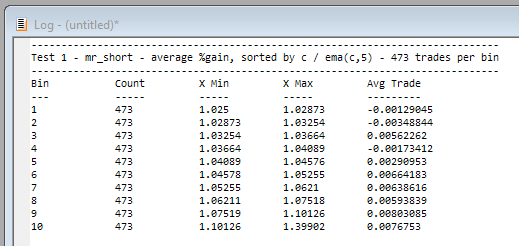The edge still appears to be there, especially at 4% or more above the EMA.

For a different view of the same factor, expectancy can be calculated for specific X intervals rather than equal counts: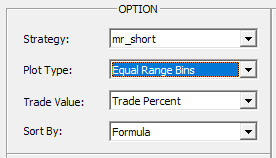...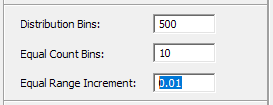Plot output: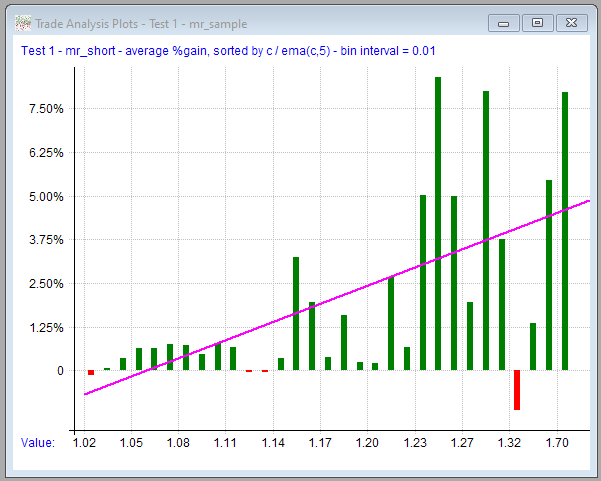Log output: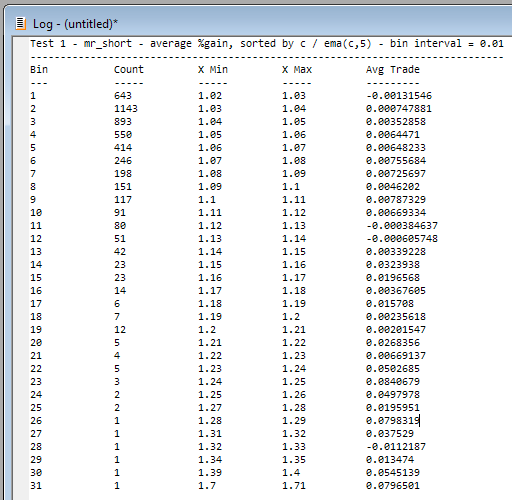Note that as you move towards the right side of the plot, each bar represents fewer data points.

For this reason I usually prefer equal-count over equal-range for bin analysis.